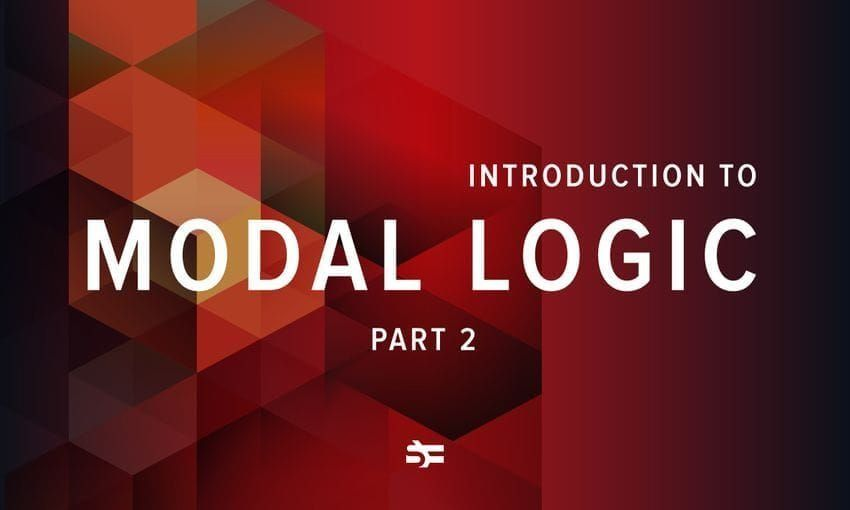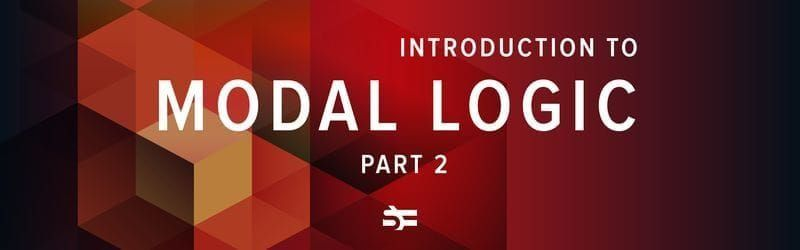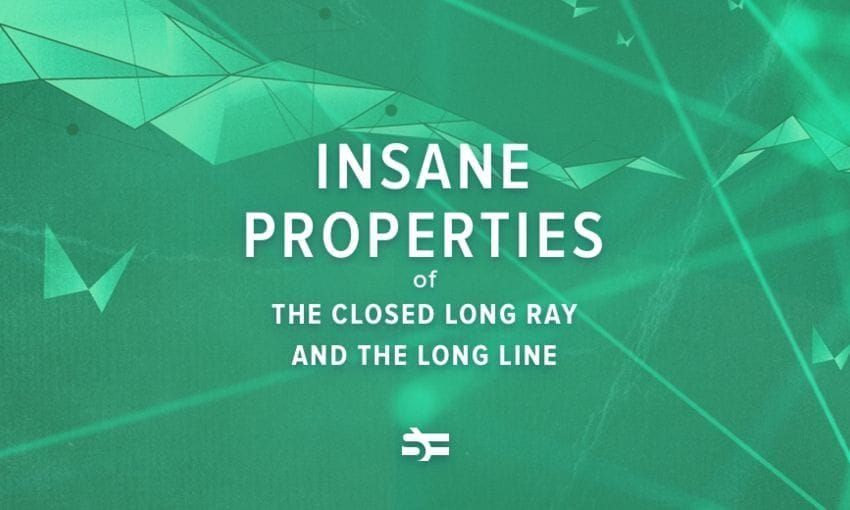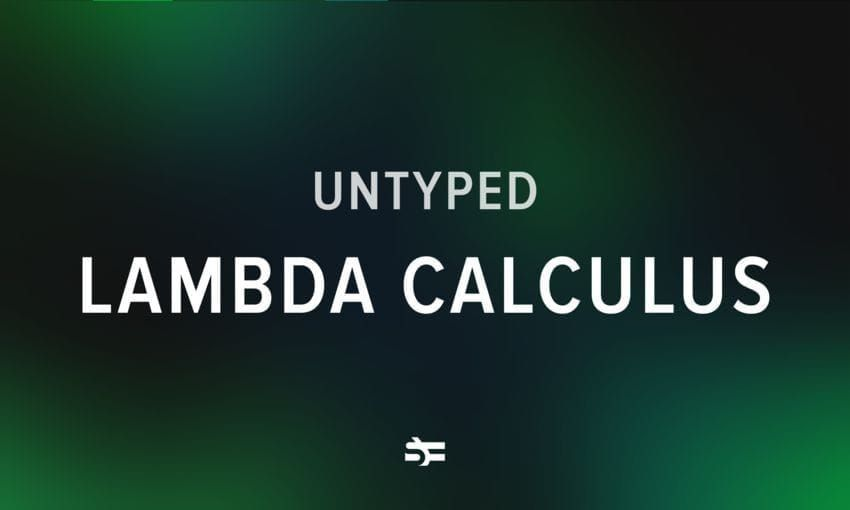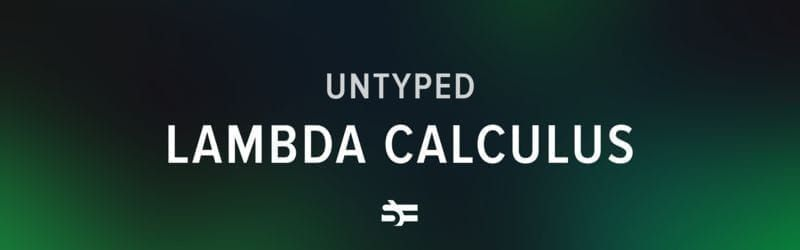# The Problem of Intermediate Recursively Enumerable Turing Degrees

###### The Turing degree of a set of natural numbers is a concept from computer science and mathematical logic that is a measure of the level of algorithmic unsolvability of the set. This post written by our friends from the ‘Mind vs Trash’ VK-community carries you deeper to the problem of the undecidable languages and the halting problem.

In computability theory, it is common to introduce the class of decidable languages first, and after that, prove that there are undecidable languages as well. There are two common ways to show it: a non-constructive proof (there is an uncountable number of subsets of $Σ*$ and there is a countable number of decidable subsets) and a constructive proof (one or another way of defining the halting problem). After that, it is common to prove that a lot of more ‘natural’ problems are undecidable, but all of them are basically reduced to the halting problem. It can be shown that the set of undecidable languages built this way is countable as well. Here a natural question appears: what about all other undecidable languages since there are so many of them?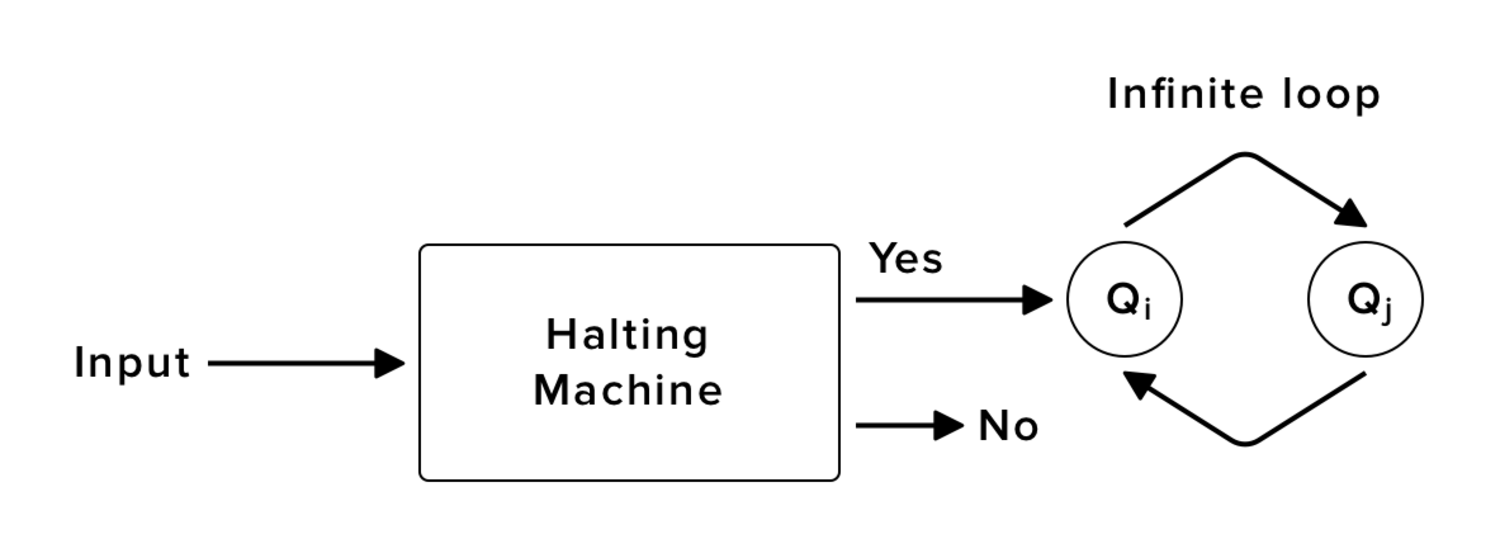For languages $A$ and $B$, $A$ is Turing reducible to $B$ ($A ≤ B$) if $A$ is decidable with an oracle for $B$. Languages are Turing equivalent ($A ≡ B$) if $A ≤ B$ and $B ≤ A$. The ≡ relation determines equivalence classes, or Turing degrees. The set of all decidable languages is one equivalence class, while the set of all undecidable languages which are Turing equivalent to the halting problem, is another. Let us try to find more classes.

Let us assume there is an oracle $O$ for the halting problem. For a program and an input, we can determine in constant time, whether the program will halt on the input. Note, that $O$ takes as input a program without an oracle, but a program that uses $O$ (in a meaningful way) cannot be rewritten without $O$ (it is not a real program as its oracle contains something non-computable), thus, such a program cannot be given as input to an oracle for determining, whether it will halt. Can it be determined, whether an arbitrary ‘program’ with such an oracle will halt ($O$ can be used for the solution as well)? The answer is: of course not. The proof is analogous to the proof of the halting problem undecidability (the proof is relativising, i.e., adding an oracle will not change it). It means, such a ‘super-halting’ problem is not Turing reducible to ‘normal’ undecidable languages, and it determines the third equivalence class.

Using an oracle for this new problem, we can build the fourth equivalence class, then the fifth, and so on. For an arbitrary Turing degree, its ‘+1’ can be defined as the Turing equivalence class of the halting problem relativised for the given Turing degree. Such an operation is called the Turing jump. It is usually denoted by a prime symbol. If the Turing degree containing decidable languages is 0, then we have the following simple case:

• Turing degree 0 (decidable languages),
• 1 = 0’ (reducible to the halting problem),
• 2 = 1’ = 0’’ (reducible to the super-halting problem),
• etc.

Is there anything in between?

Friedberg and Muchnik (1956) independently proved that there exists a pair of languages $A$ and $B$, such that both are decidable with an oracle for the halting problem, but $A$ is undecidable with an oracle for $B$, as well as $B$ is undecidable with an oracle for $A$. This result instantly complicates the structure of languages: we see that Turing degrees do not have a total order, and there are also intermediate degrees.

Today it is known that Turing degrees form a lower semilattice with a lot of interesting properties, such as:

• The cardinality of every Turing degree is countable.
• There is an uncountable number of different Turing degrees.
• For any degree $a$ there is no more than a countable number of degrees $b ≤ a$ and uncountable number of degrees $c ≥ a$.
• There are nonzero degrees without intermediate degrees between them and zero, i.e., the lattice is not dense ordered.
• For any degree $a$ there is a degree $b$ such that $a < b$ and $a′ = b′$, i.e., the Turing jump is not similar to the normal ‘+1’ operation!).
• There is an infinite sequence $a_i$ of degrees such that $a_i ≥ a_{i+1}'$.
• For every degree $a$ there is a degree strictly between $a$ and $a'$ (in fact, there is a countable sequence of pairwise incomparable degrees between $a$ and $a'$).
##### References:
1. Scott Aaronson, Quantum Computing Since Democritus, Lecture 4
2. S. Barry Cooper, Computability Theory, pp. 219-246
3. https://en.wikipedia.org/wiki/Turing_degree
4. https://en.wikipedia.org/wiki/Turing_jump
The Problem of Intermediate Recursively Enumerable Turing DegreesMore from Serokell